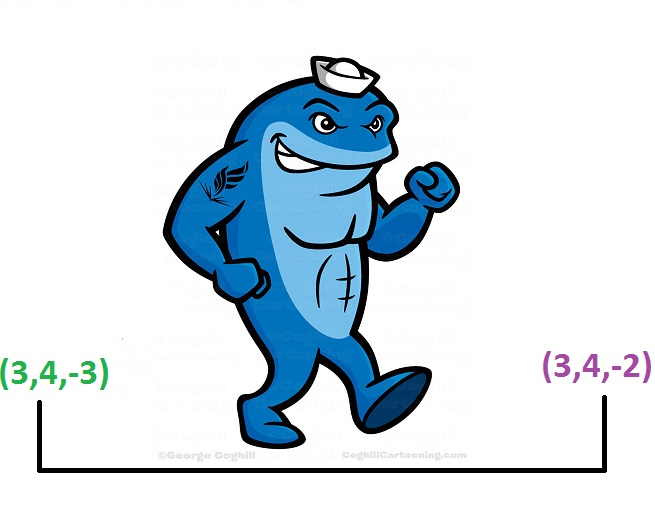# 3D Grid Walk

Probability Level 5You start at the point $(x,y,z) = (1,1,1)$ in a Cartesian coordinate system.

Every second you move randomly one unit in any of the six directions available to you.

What is the expected value for the number of seconds it will take for you to land on a point $(3l, 3m, 3n)$ where $l$, $m$ and $n$ are integers.

Clarification: You can go into any octant of the 3D Cartesian coordinate system. i.e. Any or all of $l$, $m$, and $n$ can be negative.

×